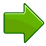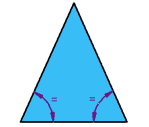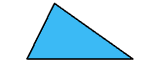# what is a   ISOSCELES TRIANGLE AND A RHOMBUS

Hi!
Here is the answer to your question.

A quadrilateral whose opposite sides are parallel and all sides are of equal lengths is called a rhombus or it may be defined as a quadrilateral having all its side congruent. Some properties of a rhombus are:

1.  Its opposite angles are of equal measure.
2.  Its diagonals are perpendicular bisectors of one another.

A triangle whose any two sides are equal is called an isosceles triangle.
The properties of isosceles triangle are:
1. Two sides of an isosceles triangle are equal.
2. Two angles (opposite to equal sides) of an isosceles triangle are equal.

Cheers!

• 1

## The Rhombusrhombus is a four-sided shape where all sides have equal length.

Also opposite sides are parallel and opposite angles are equal.

Another interesting thing is that the diagonals (dashed lines in second figure) of a rhombus bisect each other at right angles

• 0

# Triangles### A triangle has three sides and three angles## Equilateral, Isosceles and Scalene

There are three special names given to triangles that tell how many sides (or angles) are equal.

There can be 32 or no equal sides/angles:### Equilateral Triangle

Three equal sides
Three equal angles, always 60°### Isosceles Triangle

Two equal sides
Two equal angles### Scalene Triangle

No equal sides
No equal angles

• 1

Hi Pornima ,

1.) RHOMBUS :- A RHOMBUS has four sides of equal length . The opposite angles are equal and the opposite sides are parallel to each other . The diagonals of a rhombus bisect each other at right angles .

2.) TRIANGLES :- A TRIANGLE has three sides . The three angles always add to 1800 . The sum of the two sides of a triangle is always greater than the third side . There are three special names given to the triangles that tell how many sides are equal.They are Equilateral Triangle , Isosceles Triangle and Scalene Triangle.

Hope you got the answer .

Cheers !

• 0
What are you looking for?